Courses

# Graph and its representations Notes | EduRev

## Computer Science Engineering (CSE) : Graph and its representations Notes | EduRev

The document Graph and its representations Notes | EduRev is a part of the Computer Science Engineering (CSE) Course Algorithms.
All you need of Computer Science Engineering (CSE) at this link: Computer Science Engineering (CSE)

Graph and its representations

A graph is a data structure that consists of the following two components:

1. A finite set of vertices also called as nodes.
2. A finite set of ordered pair of the form (u, v) called as edge. The pair is ordered because (u, v) is not the same as (v, u) in case of a directed graph(di-graph). The pair of the form (u, v) indicates that there is an edge from vertex u to vertex v. The edges may contain weight/value/cost.
Graphs are used to represent many real-life applications: Graphs are used to represent networks. The networks may include paths in a city or telephone network or circuit network. Graphs are also used in social networks like linked In, Facebook. For example, in Facebook, each person is represented with a vertex(or node). Each node is a structure and contains information like person id, name, gender, and locale. See this for more applications of graph.

Following is an example of an undirected graph with 5 vertices: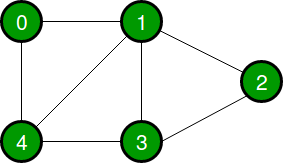The following two are the most commonly used representations of a graph.

There are other representations also like, Incidence Matrix and Incidence List. The choice of graph representation is situation-specific. It totally depends on the type of operations to be performed and ease of use.

Adjacency Matrix is a 2D array of size V x V where V is the number of vertices in a graph. Let the 2D array be adj[][], a slot adj[i][j] = 1 indicates that there is an edge from vertex i to vertex j. Adjacency matrix for undirected graph is always symmetric. Adjacency Matrix is also used to represent weighted graphs. If adj[i][j] = w, then there is an edge from vertex i to vertex j with weight w.
The adjacency matrix for the above example graph is: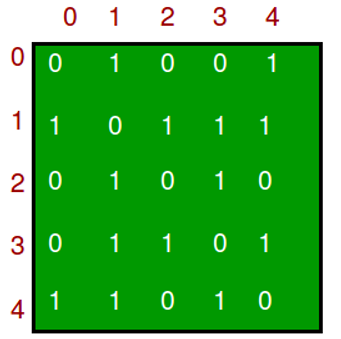Pros: Representation is easier to implement and follow. Removing an edge takes O(1) time. Queries like whether there is an edge from vertex ‘u’ to vertex ‘v’ are efficient and can be done O(1).
Cons: Consumes more space O(V2). Even if the graph is sparse(contains less number of edges), it consumes the same space. Adding a vertex is O(V2) time.

An array of lists is used. The size of the array is equal to the number of vertices. Let the array be an array[]. An entry array[i] represents the list of vertices adjacent to the ith vertex. This representation can also be used to represent a weighted graph. The weights of edges can be represented as lists of pairs. Following is the adjacency list representation of the above graph.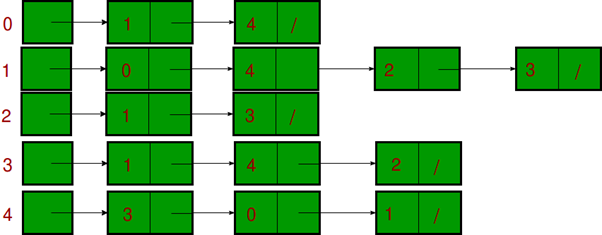Note that in the below implementation, we use dynamic arrays (vector in C++/ArrayList in Java) to represent adjacency lists instead of the linked list.

The vector implementation has advantages of cache friendliness:

• C++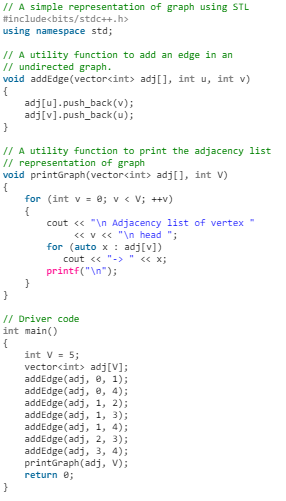• C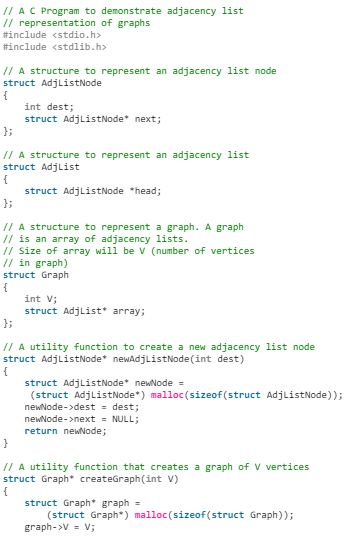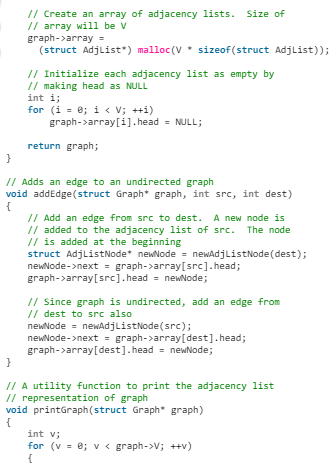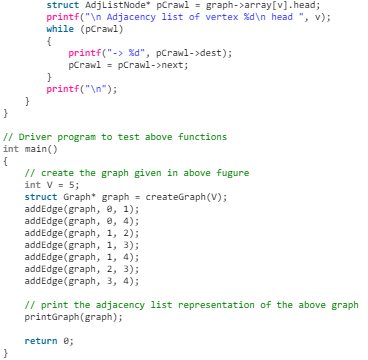• Java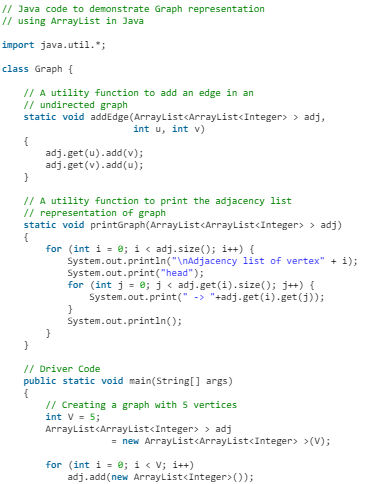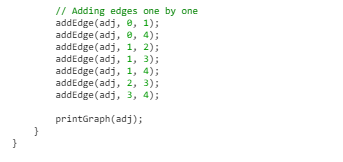• Python3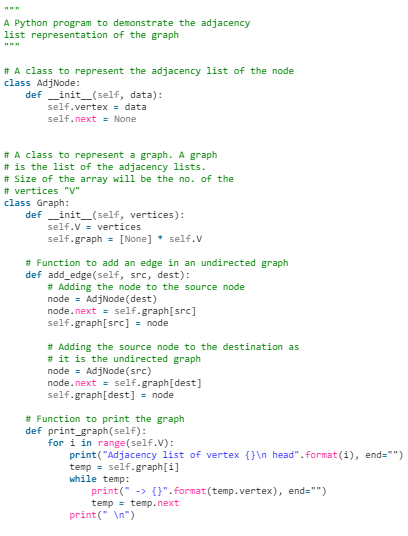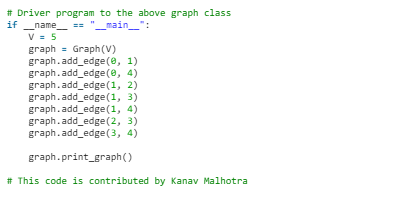• C#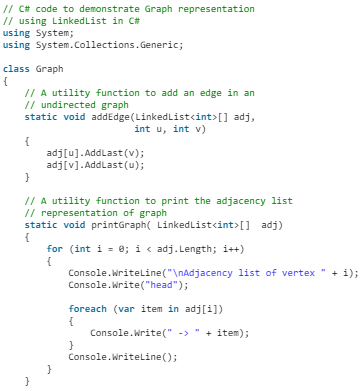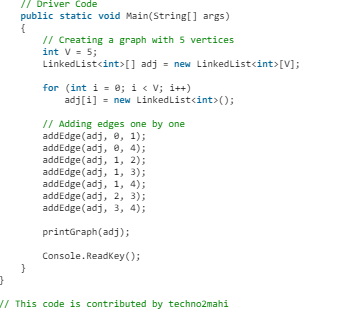Output:
head -> 0-> 2-> 3-> 4
Pros: Saves space O(|V| + |E|) . In the worst case, there can be C(V, 2) number of edges in a graph thus consuming O(V^2) space. Adding a vertex is easier.
Cons: Queries like whether there is an edge from vertex u to vertex v are not efficient and can be done O(V).

Offer running on EduRev: Apply code STAYHOME200 to get INR 200 off on our premium plan EduRev Infinity!

52 docs|31 tests

,

,

,

,

,

,

,

,

,

,

,

,

,

,

,

,

,

,

,

,

,

;